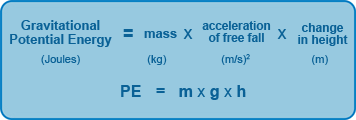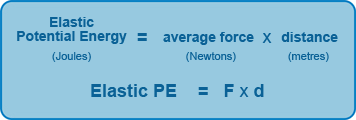## Gravitational Potential Energy

This is the energy an object possesses by reason of its position in a gravitational field. In simpler terms it can be considered as the energy an object possesses due to its height from the Earth's surface.

The formula for gravitational potential energy is:Thus the higher an object is moved from the Earth's surface the more potential energy it will possess. Like kinetic energy gravitational potential energy also depends on the mass of the object.

In order to raise an object to a certain height above the Earth's surface work is done on the object against the force of gravity. It is this worked that is done to the object that is transferred and stored as gravitational potential energy.

### Example

Calculate the gravitational potential energy of a skydiver with a mass of 80kg about to jump out of a plane at an altitude of 5000m?

Solution:

PE = m x g x h

PE = 80 x 9.8 x 5000 [remember acceleration due to gravity = 9.8 m/s2]

PE = 3920000 Joules = 3920 kilojoules (kJ)

## Elastic Potential Energy

This is the energy stored by a material or object as a result of deformation e.g. the energy stored in a bow when it is stretched.

Elastic potential energy is equal to the work done to stretch or deform the material.### Example

An Olympic archer applies a force of 100N in pulling back her bow by 0.5m. How much energy is stored in the bow?

Solution:

Elastic PE = F x d

Elastic PE = 100 x 0.5

Elastic PE = 50 Joules

Thus 50 Joules of work was done by the archer on the bow which will be transferred to the arrow when it is released.